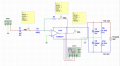# Inverting Amplifier

Joined Mar 10, 2021
2
I am stuck on the following questions with regard to an inverting amplifier. I cant seem to get my head around the questions? Can anyone help pointing me in the right direction to calculate the values required. The gain for the circuit is -100. Thanks.• Calculate gain in dB; (20log(gain) = gain in dB) dB Gain =
• Calculate the gain at cut-off frequency; fc = dB Gain -3dB =
• Calculate arithmetic gain (A) at fc; A = 10^fc dB/20 =
• Multiply Vin peak-to-peak voltage by A; Vout at fc =

Last edited by a moderator:

#### ericgibbs

Joined Jan 29, 2010
18,015
hi mani,
Welcome to AAC.
As this is Homework, please post your best attempt and the we can help.
E

Joined Mar 10, 2021
2
Hi, I have below the attempts to solve the posted problems.
Gain = 40
Gain at Cut off = 40-3=37
Gain (A) = 10^37/20 = 5x10^35
Vout at fc = 30x(5x10^35) = 1.5x10^37

Thanks

#### Audioguru again

Joined Oct 21, 2019
6,155
The gain is said to be -100 but you say it is 40. Don't you mean to say the gain is 40dB?
You say the gain at cutoff is 37 but instead it is 37dB.
37dB is 3dB less than a gain of 100 times then the answer is 70.7 times.
Vout at fc is unknown since we do not know Vin the input level or input frequency.